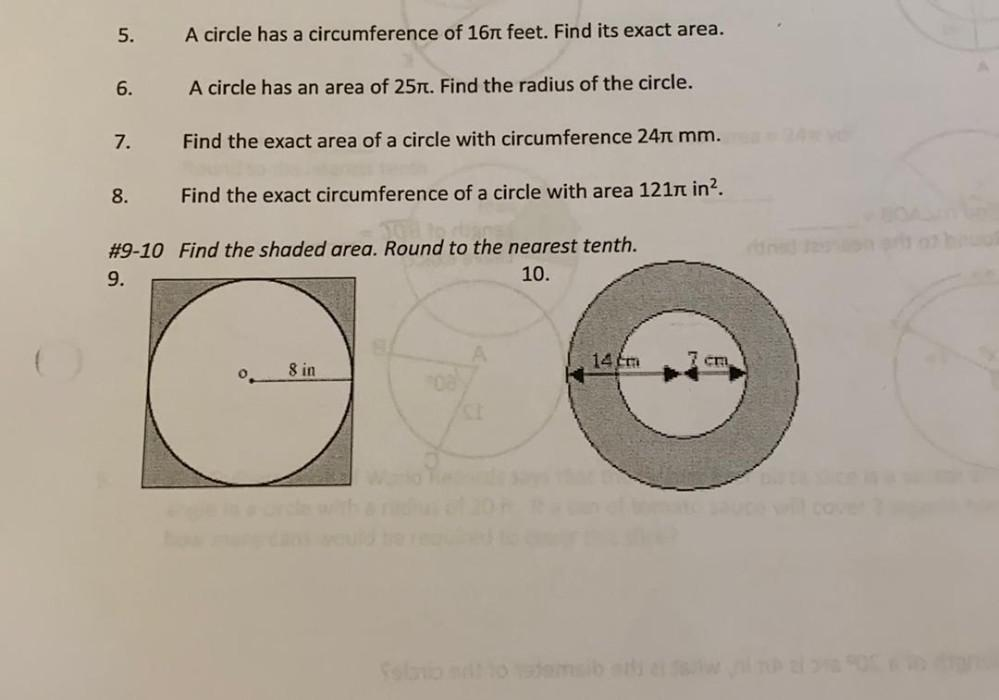Question:

# 5. A circle has a circumference of 16 feet. Find its exact area. 6. A circle has an area of 25Tt. Find the radius of the circle.5. A circle has a circumference of 16 feet. Find its exact area. 6. A circle has an area of 25Tt. Find the radius of the circle. 7. Find the exact area of a circle with circumference 24 mm. 8. Find the exact circumference of a circle with area 1217 in?. #9-10 Find the shaded area. Round to the nearest tenth. 9. 10. 1410 7 cm 8 in o